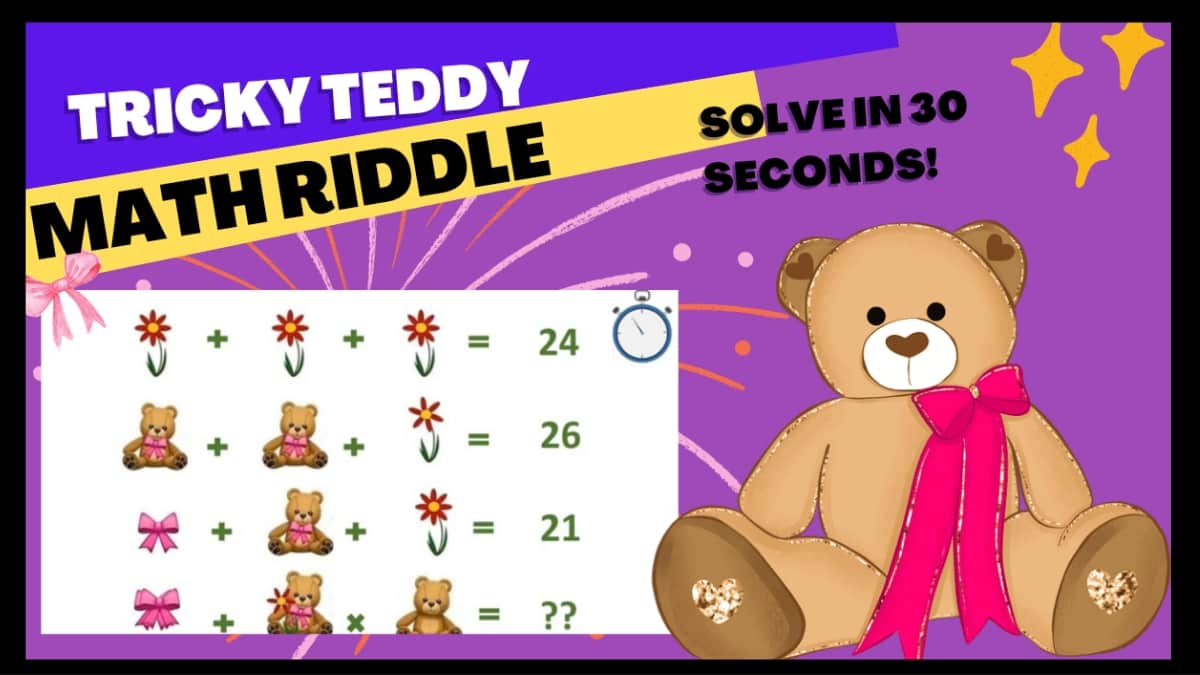# Math Riddles: Can you Solve This Tricky Teddy Puzzle In 30 Seconds? Check if you have a high IQ here!

Math Riddle Challenge: Can you solve this tricky Teddy Puzzle In 30 Seconds? Test Your IQ and sharpen your brain with this cuddly math riddle.Math Riddles: Can you Solve This Teddy Puzzle In 30 Seconds?

Math Riddle Challenge: Can you solve this Teddy Puzzle In 30 Seconds? Test Your IQ and sharpen your brain with this cuddly maths riddle.

Math riddles can make you brighter and sharper. How? Well, brain teasers are known to improve cognitive functioning and enhance your critical thinking and problem-solving abilities.

they help refresh your mind and give you a much-desired break, breaking monotony.

Look at the picture posted below you will find a math puzzle that only those with a high IQ will be able to answer.

Carefully check this math puzzle.

All the best!

Is it easy?

Were you able to solve this tricky teddy puzzle?

If you were able to solve it, let us make it a challenge.

Set a timer to 30 seconds

tick...

tock...
tick...

Time's Up!

Do you have the solution? it's ok if you don't because we do!

## Let's solve it together

As you can see the puzzle has four equations. The first one has flowers(F), the second one has teddies (T), the third one has a pretty pink bow(B) in it. The final equation has them all.

Let's Look at each.

## First Equation

We have the sum of three flowers(F) here which is 24.

### F=8

Now we know the value of the Flower is 8.

## Second Equation

Here we have the sum of two teddy bears (T )and one flower(F), which is 26.

### T=10

Now we know the value of Teddy is 10

## Third Equation

Now look at the equation very carefully, the Bow(B), Teddy(T) and the eight petal Flower (F) is 21.

### B=3

Now we know that the value of Bow is 3.

## Final Equation

There's a double bow, a teddy, and a flower with six petals merged and a teddy in this equation if you look carefully. We need to check their sum! Let's find out the missing number.

### Hence, the solution is 118

Great work!

Keep doing these math riddles and you'll be a math master in no time!

Don't miss out!

Math Riddles: Solve This Chocolate Puzzle In 30 Seconds And Test Your IQ!

Math Riddles: Can You Solve This Heart Puzzle In 30 Seconds?

Get the latest General Knowledge and Current Affairs from all over India and world for all competitive exams.
खेलें हर किस्म के रोमांच से भरपूर गेम्स सिर्फ़ जागरण प्ले पर